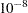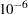The RELIABILITY Procedure
 ANALYZE Statement
<label:>ANALYZE variable <*censor-variable(values)> <=group-variables> </ options> ;
<label:>ANALYZE (variable1 variable2) <=group-variables> </ options> ;
<label:>ANALYZE variable1(variable2) <=group-variables> </ options> ;

You use the ANALYZE statement to estimate the parameters of the probability distribution specified in the DISTRIBUTION statement without producing any graphical output. The ANALYZE statement performs the same analysis as the PROBPLOT statement, but it does not produce any plots. In addition, you can use the ANALYZE statement to analyze data with the binomial and Poisson distributions. The third format for the preceding ANALYZE statement applies only to Poisson and binomial data. You can use any number of ANALYZE statements after a PROC RELIABILITY statement; each ANALYZE statement produces a separate analysis. You can specify an optional label to distinguish between multiple ANALYZE statements in the output.

You must specify one variable. If your data are right censored, you must specify a censor-variable and, in parentheses, the values of the censor-variable that correspond to censored data values.

If you are using the binomial or Poisson distributions, you must specify variable1 to represent a binomial or Poisson count and variable2 to provide an exposure measure for the Poisson distribution or the binomial sample size for the binomial distribution.

You can optionally specify one or two group-variables. The ANALYZE statement produces an analysis for each level combination of the group-variable values. The observations in a given level are referred to as a cell.

The elements of the ANALYZE statement are described as follows.

variable

represents the data for which an analysis is to be produced. A variable must be a numeric variable in the input data set.

censor-variable(values)

indicates which observations in the input data set are right censored. You specify the values of censor-variable that represent censored observations by placing those values in parentheses after the variable name. If your data are not right censored, then you omit the specification of censor-variable; otherwise, censor-variable must be a numeric variable in the input data set.

(variable1 variable2)

is another method of specifying the data. You can use this syntax in a situation where uncensored, interval-censored, left-censored, and right-censored values occur in the same set of data. Table 12.26 shows how you use this syntax to specify different types of censoring by using combinations of missing and nonmissing values. See the section Lognormal Analysis with Arbitrary Censoring for an example of using this syntax to create a probability plot.

variable1

represents the count data for which a Poisson or binomial analysis is to be produced. A variable1 must be a numeric variable in the input data set.

variable2

provides either an exposure measure for a Poisson analysis or a binomial number of trials for a binomial analysis. A variable2 must be a numeric variable in the input data set.

group-variables

are one or two group variables. If no group variables are specified, a single analysis is produced. The group-variables can be numeric or character variables in the input data set.

Note that the parentheses surrounding the textitgroup-variables are needed only if two group variables are specified.

options

control the features of the analysis. All options are specified after a slash (/) in the ANALYZE statement.

Summary of Options

The following tables summarize the options available in the ANALYZE statement. You can specify one or more of these options to control the parameter estimation and provide optional analyses.

Table 12.4 Analysis Options for Distributions Other Than Poisson or Binomial

Option

Option Description

CONFIDENCE=number

specifies the confidence coefficient for all confidence intervals. Specify a number between 0 and 1. The default value is 0.95.

CONVERGE=number

specifies the convergence criterion for maximum likelihood fit. See the section Maximum Likelihood Estimation for details.

CONVH=number

specifies the convergence criterion for the relative Hessian convergence criterion. See the section Maximum Likelihood Estimation for details.

CORRB

requests parameter correlation matrix

COVB

requests parameter covariance matrix

FITTYPE | FIT=

specifies method of estimating distribution parameters

LSYX

-least squares fit to the probability plot. The probability axis is the dependent variable.

| LSXY

-least squares fit to the probability plot. The lifetime axis is the dependent variable.

| MLE

-maximum likelihood (default)

| NONE

-no fit is computed

| WEIBAYES

-Weibayes

<(CONFIDENCE|CONF=number)>

number is the confidence coefficient for the Weibayes fit and is between 0 and 1. The default is 0.95.

ITPRINT

requests iteration history for maximum likelihood fit

ITPRINTEM

requests iteration history for the Turnbull algorithm

LRCL

requests likelihood ratio confidence intervals for distribution parameters

LRCLPER

requests likelihood ratio confidence intervals for distribution percentiles

LOCATION=number <LINIT>

specifies fixed or initial value of location parameter

MAXIT=number

specifies maximum number of iterations allowed for maximum likelihood fit

MAXITEREM |

MAXITEM=number1 <,number2>

number1 specifies maximum number of iterations allowed for Turnbull algorithm. Iteration history will be printed in increments of number2 if requested with ITPRINTEM. See the section Interval-Censored Data for details.

NOPCTILES

suppress computation of percentiles

NOPOLISH

suppress setting small interval probabilities to zero in Turnbull algorithm. See the section Interval-Censored Data for details.

PCTLIST=number-list

specifies list of percentages for which to compute percentile estimates. number-list must be a list of numbers separated by blanks or commas. Each number in the list must be between 0 and 100.

PPOS=

specifies plotting position type. See the section Probability Plotting for details.

EXPRANK

-expected ranks

| MEDRANK

-median ranks

| MEDRANK1

-median ranks (exact formula)

| KM

-Kaplan-Meier

| MKM

-modified Kaplan-Meier (default)

| (NA | NELSONAALEN)

- Nelson-Aalen

PPOUT

requests table of cumulative probabilities

PRINTPROBS

print intervals and associated probabilities for the Turnbull algorithm

PROBLIST=number-list

specifies list of initial values for Turnbull algorithm. See the section Interval-Censored Data for details.

PSTABLE=number

specifies stable parameterization. The number must be between zero and one. See the section Stable Parameters for further information.

SCALE=number <SCINIT>

specifies fixed or initial value of scale parameter

SHAPE=number <SHINIT>

specifies fixed or initial value of shape parameter

SINGULAR=number

specifies singularity criterion for matrix inversion

SURVTIME=number-list

requests that the survival function, cumulative distribution function, and confidence limits be computed for values in number-list. See the section Reliability Function for details.

THRESHOLD=number

specifies a fixed threshold parameter. See Table 12.49 for the distributions with a threshold parameter.

TOLLIKE=number

specifies criterion for convergence in the Turnbull algorithm. Default is. See the section Interval-Censored Data for details.

TOLPROB=number

specifies criterion for setting interval probability to zero in the Turnbull algorithm. Default is. See the section Interval-Censored Data for details.

Table 12.5 Analysis Options for Poisson and Binomial Distributions

Option

Option Description

CONFIDENCE=number

specifies the confidence coefficient for all confidence intervals. Specify a number between 0 and 1. The default value is 0.95.

PREDICT(number)

requests predicted counts for exposure number for Poisson or sample size number for binomial

TOLERANCE(number)

requests exposure for Poisson or sample size for binomial to estimate Poisson rate or binomial probability within number with probability given by the CONFIDENCE= optionPrevious Page | Next Page | Top of Page